# MCAT Physical : Other Principles Governing Electrons

## Example Questions

### Example Question #61 : Nuclear Chemistry And Electrons

Which of these statement is true regarding an emission spectrum?

The emission and absorption spectrum of an element show different wavelengths

It is not possible to classify elements by their emission spectra because the spectra overlap so extensively

As elements get heavier, their increasingly complex emission spectrum is simply added to the emission spectrum of the lighter elements

The Hubble space telescope would observe absorption spectra from stars

The emission spectrum of each element is unique

The emission spectrum of each element is unique

Explanation:

When energy is added to an atom, usually in the form of heat, the electrons may be displaced to higher quantum states; it is said that they "jump" to new orbital states. When they fall back to unexcited, or ground states, they emit exactly the amount of energy they took absorbed, either all at once, or in steps.

This emission is discrete, meaning that it creates light at very specific wavelengths. The emission spectrum is completely unique for each elemental atom, and the wavelengths are identical in the emission and absorption spectra, which can be thought of as photographic positive and negative images of exactly the same phenomenon.

### Example Question #1 : Other Principles Governing Electrons

Suppose an electron is excited from its ground state to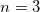. Which of the following effects is most likely to occur?

A photon is emitted

The electron increases in velocity

The electron decreases in velocity

A photon is absorbed

A photon is absorbed

Explanation:

This question is testing your knowledge of what happens when an electron is excited. Electron excitation always accompanies an increase in energy, the opposite of which is equally true for electrons that fall back toward the ground state. Electron energy transitions are accompanied by electromagnetic energy consumption or release. When an electron increases in energy, it absorbs a certain unit of electromagnetic energy: a photon. When the electron decreases in energy, it emits the lost energy by releasing a photon.

### Example Question #3 : Other Principles Governing Electrons

Photons can be generated with different wavelengths, depending on the number of energy levels available. What is the minimum number of different energy levels needed to produce six different wavelengths of light?

Two

Six

Four

Three

Seven

Four

Explanation:

From Bohr's model of the hydrogen atom, we know that as electrons jump to a higher energy state they become more excited, and absorb more energy. Additionally, we know that when an electron moves from a higher energy state to a lower energy state, it releases energy in the form of a photon. Because the emitted photon can be characterized by its wavelength, each jump between two different energy states will have a unique and different wavelength.

To produce six different wavelengths, we would need four different energy levels: n=1, n=2, n=3, n=4. The different wavelengths produced could be described by the following energy transitions.

1) 4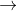1

2) 42

3) 43

4) 32

5) 31

6) 21

### Example Question #4 : Other Principles Governing Electrons

Electronegativity is an important concept in physical chemistry, and often used to help quantify the dipole moment of polar compounds. Polar compounds are different from those compounds that are purely nonpolar or purely ionic. An example can be seen by contrasting sodium chloride, NaCl, with an organic molecule, R-C-OH. The former is purely ionic, and the latter is polar covalent.

When comparing more than one polar covalent molecule, we use the dipole moment value to help us determine relative strength of polarity. Dipole moment, however, is dependent on the electronegativity of the atoms making up the bond. Electronegativity is a property inherent to the atom in question, whereas dipole moment is a property of the bond between them.

For example, oxygen has an electronegativity of 3.44, and hydrogen of 2.20. In other words, oxygen more strongly attracts electrons when in a bond with hydrogen. This leads to the O-H bond having a dipole moment.

When all the dipole moments of polar bonds in a molecule are summed, the molecular dipole moment results, as per the following equation.

Dipole moment = charge * separation distance

A scientist undertakes an effort to visualize the electron cloud that makes up the single O-H bond. You tell him that this will be complicated by the fact that the more you know about an electron's momentum, the less you know about its position, and vice versa. What principle are you describing?

Principle of Electron Delocalization

Aufbau Principle

Hund's Rule

Heisenberg Uncertainty Principle

Principle of Subatomic Probability

Heisenberg Uncertainty Principle

Explanation:

The Heisenberg Uncertainty Principle tells us exactly this. At the quantum level, the more you know about a body's momentum, the less you know about its position.

### Example Question #63 : Nuclear Chemistry And Electrons

Light waves are known to exist in a wave-particle duality. When objects approach the speed of light, this duality supposedly extends to other forms of matter. A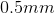lead pencil point weighs roughly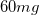. If all of this mass was converted into wave energy, how much energy would be released?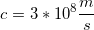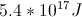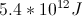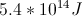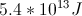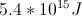Explanation:

This is a problem utilizing Einstein's equation: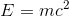Before we can solve for the energy, we need to convert the mass to kilograms.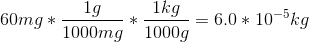Use this mass and the speed of light to solve for the energy.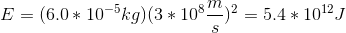### Example Question #4 : Other Principles Governing Electrons

Which of the following statements are true when there are two electrons in the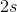subshell?

One electron will be emitted

One electron will be absorbed

They must be occupying different orbitals

They must have opposite spins

They must be oppositely charged